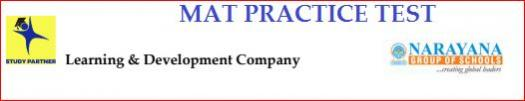# NTSE MAT Part Test 3

50 Questions | Total Attempts: 91SettingsInstructionsThe Test (Mental Ability) consists of 50 Questions (Q. Nos. 1 to 50)All questions carry one mark each.

• 1.
In the following diagram the boys wh​o are athletic and are disciplined are indicated by which number?
• A.

1

• B.

2

• C.

10

• D.

6

• 2.
Study the diagram given below and answer each of the following questions.How many persons who take tea and wine but not coffee ?
• A.

20

• B.

17

• C.

25

• D.

15

• 3.
Find out the alternative figure which contains figure (X) as its part.
• A.

1

• B.

2

• C.

3

• D.

4

• 4.
Find out the alternative figure which contains figure (X) as its part.
• A.

1

• B.

2

• C.

3

• D.

4

• 5.
Find out the alternative figure which contains figure (X) as its part.
• A.

1

• B.

2

• C.

3

• D.

4

• 6.
Find out the alternative figure which contains figure (X) as its part.
• A.

1

• B.

2

• C.

3

• D.

4

• 7.
Find out the alternative figure which contains figure (X) as its part.
• A.

1

• B.

2

• C.

3

• D.

4

• 8.
Find out the alternative figure which contains figure (X) as its part.
• A.

1

• B.

2

• C.

3

• D.

4

• 9.
​Find out the alternative figure which contains figure (X) as its part.
• A.

1

• B.

2

• C.

3

• D.

4

• 10.
Find out the alternative figure which contains figure (X) as its part.
• A.

Option 1

• B.

Option 2

• C.

Option 3

• D.

Option 4

• 11.
Find out the alternative figure which contains figure (X) as its part.
• A.

1

• B.

2

• C.

3

• D.

4

• 12.
A,B,C,D,E,F,G,H are sitting on a merry-go-round facing at the centre.
• D is second to the left on H who is third to the left of A.
• B is fourth to the right of C who is immediate neighbour of H.
• G is not a neighbour of B or C.
• F is not a neighbour of B.
​Who is third to the left of B?
• A.

F

• B.

A

• C.

E

• D.

G

• 13.
Amongst five friends, Lata, Alka, Rani, Asha and Sadhana. Lata is older than only three of her friends. Alka is younger to Asha and Lata. Rani is older than only Sadhana.Who amongst them is the eldest ?
• A.

Asha

• B.

Lata

• C.

Alka

• D.

• 14.
A, B, C, D and E are sitting on a bench. A is sitting next to B, C is sitting next to D, D is not sitting next to E,who is sitting on the left end of the bench. C is on the second position from the Fight. A is to the right of Band E.Counting from the left, in which position is A sitting?
• A.

2

• B.

3

• C.

5

• D.

Cannot be determined from the given conditions

• 15.
Direction : When the signs are changed as shown below, which one of the given equations will be correct?
• A.

Option 1

• B.

Option 2

• C.

Option 3

• D.

Option 4

• 16.
How many triangles are there in the given figure?
• A.

15

• B.

14

• C.

13

• D.

11

• 17.
How many quadrilaterals are ,there in the given figure?
• A.

7

• B.

8

• C.

9

• D.

10

• 18.
How many semi circles are there in the given figure,?
• A.

16

• B.

18

• C.

20

• D.

24

• 19.
Directions : The following questions are based on the given intersecting figures.Which pair of numbers given below are inside any two figures only?
• A.

9, 12

• B.

3, 11

• C.

3,13

• D.

11, 1

• 20.
Directions : The following questions are based on the given intersecting figures. Which of the following number lies in the figures II, III and IV only?
• A.

9

• B.

5

• C.

8

• D.

10

• 21.
Which one of the following Venn diagrams represents the relationshipamong A, B, C and D under theconditions given below:a) All or C belongs to B. b) Some part of B belongs to A.c) Some parts of both C and B belong to D.
• A.

Option 1

• B.

Option 2

• C.

Option 3

• D.

Option 4

• 22.
If A + B = 2C and C + D = 2A, then
• A.

A + C = B + D

• B.

A + C = 2D

• C.

A + D = B + C

• D.

A + C = 2B

• 23.
Given interchanges : Signs + and - , numbers 4 and 8.
• A.

4 + 8 - 12 = 12

• B.

4 - 8 + 12 = 0

• C.

8 + 4 - 12 = 24

• D.

8 - 4 + 12 = 8

• 24.
Find out the two signs to be interchanged for making following equation correct5 + 3 x 8 - 12 / 4 =3
• A.

+ and -

• B.

- and /

• C.

+ and x

• D.

+ and /

• 25.
If × stands for 'addition', ÷ stands for 'subtraction', + stands for 'multiplication' and-stands for 'division', then 20 × 8 ÷ 8 - 4 + 2 = ?
• A.

80

• B.

25

• C.

24

• D.

5Back to top